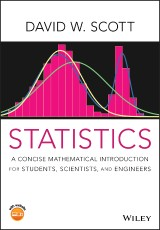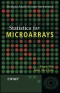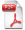# Details## Statistics

A Concise Mathematical Introduction for Students, Scientists, and Engineers
1. Aufl.
 von: David W. Scott 40,99 € Verlag: Wiley Format: PDF Veröffentl.: 20.07.2020 ISBN/EAN: 9781119675815 Sprache: englisch Anzahl Seiten: 180

DRM-geschütztes eBook, Sie benötigen z.B. Adobe Digital Editions und eine Adobe ID zum Lesen.

## Beschreibungen

#### Titelbeschreibung

<p><i>Statistic: A Concise Mathematical Introduction for Students and Scientists </i>offers a one academic term text that prepares the student to broaden their skills in statistics, probability and inference, prior to selecting their follow-on courses in their chosen fields, whether it be engineering, computer science, programming, data sciences, business or economics. </p> <p>The book places focus early on continuous measurements, as well as discrete random variables. By invoking simple and intuitive models and geometric probability, discrete and continuous experiments and probabilities are discussed throughout the book in a natural way. Classical probability, random variables, and inference are discussed, as well as material on understanding data and topics of special interest.<br /> <br /> Topics discussed include:</p> <p>•      Classical equally likely outcomes</p> <p>•      Variety of models of discrete and continuous probability laws</p> <p>•      Likelihood function and ratio</p> <p>•      Inference</p> <p>•      Bayesian statistics<br /><br /></p> <p>With the growth in the volume of data generated in many disciplines that is enabling the growth in data science, companies now demand statistically literate scientists and this textbook is the answer, suited for undergraduates studying science or engineering, be it computer science, economics, life sciences, environmental, business, amongst many others. Basic knowledge of bivariate calculus, R language, Matematica and JMP is useful, however there is an accompanying website including sample R and Mathematica code to help instructors and students.</p>

#### Inhaltsverzeichnis

<p>Preface xiii</p> <p><b>1 Data Analysis and Understanding </b><b>1</b></p> <p>1.1 Exploring the Distribution of Data 1</p> <p>1.1.1 Pearson’s Father–Son Height Data 2</p> <p>1.1.2 Lord Rayleigh’s Data 3</p> <p>1.1.3 Discussion 4</p> <p>1.2 Exploring Prediction Using Data 4</p> <p>1.2.1 Body and Brain Weights of Land Mammals 5</p> <p>1.2.2 Space Shuttle Flight 25 5</p> <p>1.2.3 Pearson’s Father–Son Height Data Revisited 7</p> <p>1.2.4 Discussion 8</p> <p>Problems 8</p> <p><b>2 Classical Probability </b><b>11</b></p> <p>2.1 Experiments with Equally Likely Outcomes 11</p> <p>2.1.1 Simple Outcomes 12</p> <p>2.1.2 Compound Events and Set Operations 12</p> <p>2.2 Probability Laws 13</p> <p>2.2.1 Union and Intersection of Events <i>A </i>and <i>B </i>13</p> <p>2.2.1.1 Case (i) 14</p> <p>2.2.1.2 Cases (ii) and (iii) 14</p> <p>2.2.1.3 Case (iv) 14</p> <p>2.2.2 Conditional Probability 14</p> <p>2.2.2.1 Definition of Conditional Probability 15</p> <p>2.2.2.2 Conditional Probability With More Than Two Events 15</p> <p>2.2.3 Independent Events 16</p> <p>2.2.4 Bayes Theorem 17</p> <p>2.2.5 Partitions and Total Probability 18</p> <p>2.3 Counting Methods 19</p> <p>2.3.1 With Replacement 20</p> <p>2.3.2 Without Replacement (Permutations) 20</p> <p>2.3.3 Without Replacement or Order (Combinations) 21</p> <p>2.3.4 Examples 21</p> <p>2.3.5 Extended Combinations (Multinomial) 22</p> <p>2.4 Countable Sets: Implications as <i>n </i>→ ∞ 22</p> <p>2.4.1 Selecting Even or Odd Integers 22</p> <p>2.4.2 Selecting Rational Versus Irrational Numbers 23</p> <p>2.5 Kolmogorov’s Axioms 23</p> <p>2.6 Reliability: Series Versus Parallel Networks 24</p> <p>2.6.1 Series Network 24</p> <p>2.6.2 Parallel Network 25</p> <p>Problems 26</p> <p><b>3 Random Variables and Models Derived From Classical Probability and Postulates </b><b>27</b></p> <p>3.1 Random Variables and Probability Distributions: Discrete Uniform Example 27</p> <p>3.1.1 Toss of a Single Die 28</p> <p>3.1.2 Toss of a Pair of Dice 28</p> <p>3.2 The Univariate Probability Density Function: Continuous Uniform Example 30</p> <p>3.2.1 Using the PDF to Compute Probabilities 32</p> <p>3.2.2 Using the PDF to Compute Relative Odds 33</p> <p>3.3 Summary Statistics: Central and Non-Central Moments 33</p> <p>3.3.1 Expectation, Average, and Mean 34</p> <p>3.3.2 Expectation as a Linear Operator 35</p> <p>3.3.3 The Variance of a Random Variable 35</p> <p>3.3.4 Standardized Random Variables 36</p> <p>3.3.5 Higher Order Moments 37</p> <p>3.3.6 Moment Generating Function 37</p> <p>3.3.7 Measurement Scales and Units of Measurement 38</p> <p>3.3.7.1 The Four Measurement Scales 38</p> <p>3.3.7.2 Units of Measurement 39</p> <p>3.4 Binomial Experiments 39</p> <p>3.5 Waiting Time for a Success: Geometric PMF 42</p> <p>3.6 Waiting Time for <i>r </i>Successes: Negative Binomial 43</p> <p>3.7 Poisson Process and Distribution 43</p> <p>3.7.1 Moments of the Poisson PMF 44</p> <p>3.7.2 Examples 45</p> <p>3.8 Waiting Time for Poisson Events: Negative Exponential PDF 45</p> <p>3.9 The Normal Distribution (Also Known as the Gaussian Distribution) 46</p> <p>3.9.1 Standard Normal Distribution 48</p> <p>3.9.2 Sums of Independent Normal Random Variables 49</p> <p>3.9.3 Normal Approximation to the Poisson Distribution 49</p> <p>Problems 50</p> <p><b>4 Bivariate Random Variables, Transformations, and Simulations </b><b>51</b></p> <p>4.1 Bivariate Continuous Random Variables 51</p> <p>4.1.1 Joint CDF and PDF Functions 51</p> <p>4.1.2 Marginal PDF 52</p> <p>4.1.3 Conditional Probability Density Function 52</p> <p>4.1.4 Independence of Two Random Variables 54</p> <p>4.1.5 Expectation, Correlation, and Regression 54</p> <p>4.1.5.1 Covariance and Correlation 55</p> <p>4.1.5.2 Regression Function 55</p> <p>4.1.6 Independence of <i>n </i>Random Variables 56</p> <p>4.1.7 Bivariate Normal PDF 56</p> <p>4.1.8 Correlation, Independence, and Confounding Variables 57</p> <p>4.2 Change of Variables 57</p> <p>4.2.1 Examples: Two Uniform Transformations 57</p> <p>4.2.2 One-Dimensional Transformations 58</p> <p>4.2.2.1 Example 1: Negative exponential PDF 59</p> <p>4.2.2.2 Example 2: Cauchy PDF 60</p> <p>4.2.2.3 Example 3: Chi-squared PDF with one degree of freedom 60</p> <p>4.2.3 Two-Dimensional Transformations 60</p> <p>4.3 Simulations 62</p> <p>4.3.1 Generating Uniform Pseudo-Random Numbers 62</p> <p>4.3.1.1 Reproducibility 62</p> <p>4.3.1.2 RANDU 62</p> <p>4.3.2 Probability Integral Transformation 63</p> <p>4.3.3 Event-driven Simulation 63</p> <p>Problems 64</p> <p><b>5 Approximations and Asymptotics </b><b>67</b></p> <p>5.1 Why Do We Like Random Samples? 67</p> <p>5.1.1 When <i>u</i>(<i>X</i>) Takes a Product Form 68</p> <p>5.1.2 When <i>u</i>(<i>X</i>) Takes a Summation Form 68</p> <p>5.2 Useful Inequalities 69</p> <p>5.2.1 Markov’s Inequality 69</p> <p>5.2.2 Chebyshev’s Inequality 70</p> <p>5.2.3 Jensen’s Inequality 70</p> <p>5.2.4 Cauchy–Schwarz Inequality 71</p> <p>5.3 Sequences of Random Variables 72</p> <p>5.3.1 Weak Law of Large Numbers 73</p> <p>5.3.2 Consistency of the Sample Variance 73</p> <p>5.3.3 Relationships Among the Modes of Convergence 74</p> <p>5.3.3.1 Proof of Result (5.21) 74</p> <p>5.3.3.2 Proof of Result (5.22) 74</p> <p>5.4 Central Limit Theorem 75</p> <p>5.4.1 Moment Generating Function for Sums 75</p> <p>5.4.2 Standardizing the Sum <i>S<sub>n</sub> </i>75</p> <p>5.4.3 Proof of Central Limit Theorem 76</p> <p>5.5 Delta Method and Variance-stabilizing Transformations 77</p> <p>Problems 78</p> <p><b>6 Parameter Estimation </b><b>79</b></p> <p>6.1 Desirable Properties of an Estimator 80</p> <p>6.2 Moments of the Sample Mean and Variance 80</p> <p>6.2.1 Theoretical Mean and Variance of the Sample Mean 81</p> <p>6.2.2 Theoretical Mean of the Sample Variance 81</p> <p>6.2.3 Theoretical Variance of the Sample Variance 82</p> <p>6.3 Method of Moments (MoM) 82</p> <p>6.4 Sufficient Statistics and Data Compression 83</p> <p>6.5 Bayesian Parameter Estimation 85</p> <p>6.6 Maximum Likelihood Parameter Estimation 86</p> <p>6.6.1 Relationship to Bayesian Parameter Estimation 87</p> <p>6.6.2 Poisson MLE Example 87</p> <p>6.6.3 Normal MLE Example 87</p> <p>6.6.4 Uniform MLE Example 88</p> <p>6.7 Information Inequalities and the Cramér–Rao Lower Bound 89</p> <p>6.7.1 Score Function 89</p> <p>6.7.2 Asymptotics of the MLE 90</p> <p>6.7.3 Minimum Variance of Unbiased Estimators 91</p> <p>6.7.4 Examples 91</p> <p>Problems 92</p> <p><b>7 Hypothesis Testing </b><b>93</b></p> <p>7.1 Setting up a Hypothesis Test 94</p> <p>7.1.1 Example of a Critical Region 94</p> <p>7.1.2 Accuracy and Errors in Hypothesis Testing 95</p> <p>7.2 Best Critical Region for Simple Hypotheses 96</p> <p>7.2.1 Simple Example Continued 96</p> <p>7.2.2 Normal Shift Model with Common Variance 97</p> <p>7.3 Best Critical Region for a Composite Alternative Hypothesis 98</p> <p>7.3.1 Negative Exponential Composite Hypothesis Test 99</p> <p>7.3.1.1 Example 99</p> <p>7.3.1.2 Alternative Critical Regions 99</p> <p>7.3.1.3 Mount St. Helens Example 100</p> <p>7.3.2 Normal Shift Model with Common But Unknown Variance: The <i>T</i>-test 102</p> <p>7.3.3 The Random Variable <i>T<sub>n</sub></i><sub>−1 </sub>102</p> <p>7.3.3.1 Where We Show <i>X </i>and <i>S</i><sup>2</sup> are Independent 102</p> <p>7.3.3.2 Where We Show That <i>S</i><sup>2</sup> Scaled is <i>𝜒</i><sup>2</sup>(<i>n </i>− 1) 103</p> <p>7.3.3.3 Where We Finally Derive the <i>T </i>PDF 103</p> <p>7.3.4 The One-Sample <i>T</i>-test 104</p> <p>7.3.5 Example 105</p> <p>7.3.6 Other <i>T</i>-tests 106</p> <p>7.3.6.1 Paired <i>T</i>-test 106</p> <p>7.3.6.2 Two-Sample <i>T</i>-test 107</p> <p>7.3.6.3 Example Two-Sample <i>T</i>-test: Lord Rayleigh’s Data 107</p> <p>7.4 Reporting Results: <i>p</i>-values and Power 108</p> <p>7.4.1 Example When the Null Hypothesis Is Rejected 109</p> <p>7.4.2 When the Null Hypothesis is Not Rejected 109</p> <p>7.4.3 The Power Function 110</p> <p>7.5 Multiple Testing and the Bonferroni Correction 111</p> <p>Problems 111</p> <p><b>8 Confidence Intervals and Other Hypothesis Tests </b><b>113</b></p> <p>8.1 Confidence Intervals 113</p> <p>8.1.1 Confidence Interval for <i>𝜇</i>: Normal Data, <i>𝜎</i><sup>2</sup> Known 113</p> <p>8.1.2 Confidence Interval for <i>𝜇</i>: <i>𝜎</i><sup>2</sup> Unknown 114</p> <p>8.1.3 Confidence Intervals and <i>p</i>-values 115</p> <p>8.2 Hypotheses About the Variance and the <i>F</i>-Distribution 115</p> <p>8.2.1 The <i>F</i>-Distribution 116</p> <p>8.2.2 Hypotheses About the Value of the Variance 116</p> <p>8.2.3 Confidence Interval for the Variance 117</p> <p>8.2.4 Two-Sided Alternative for Testing <i>𝜎</i><sup>2</sup> = <i>𝜎</i><sup>2</sup> <sub>0</sub> 117</p> <p>8.3 Pearson’s Chi-Squared Tests 118</p> <p>8.3.1 The Multinomial PMF 118</p> <p>8.3.2 Goodness-of-Fit (GoF) Tests 118</p> <p>8.3.3 Two-Category Binomial Case 119</p> <p>8.3.4 <i>m</i>-Category Multinomial Case 120</p> <p>8.3.5 Goodness-of-Fit Test for a Parametric Model 120</p> <p>8.3.6 Tests for Independence in Contingency Tables 122</p> <p>8.4 Correlation Coefficient Tests and CIs 123</p> <p>8.4.1 How to Test if the Correlation <i>𝜌 </i>= 0 123</p> <p>8.4.2 Confidence Intervals and Tests for a General Correlation Coefficient 125</p> <p>8.5 Linear Regression 125</p> <p>8.5.1 Least Squares Regression 125</p> <p>8.5.2 Distribution of the Least-Squares Parameters 126</p> <p>8.5.3 A Confidence Interval for the Slope 127</p> <p>8.5.4 A Two-side Hypothesis Test for the Slope 128</p> <p>8.5.5 Predictions at a New Value 128</p> <p>8.5.6 Population Interval at a New Value 128</p> <p>8.6 Analysis of Variance 129</p> <p>Problems 131</p> <p><b>9 Topics in Statistics </b><b>133</b></p> <p>9.1 MSE and Histogram Bin Width Selection 133</p> <p>9.1.1 MSE Criterion for Biased Estimators 133</p> <p>9.1.2 Case Study: Optimal Histogram Bin Widths 134</p> <p>9.1.3 Examples with Normal Data 137</p> <p>9.1.4 Normal Reference Rules for the Histogram Bin Width 137</p> <p>9.1.4.1 Scott’s Rule 137</p> <p>9.1.4.2 Freedman–Diaconis Rule 137</p> <p>9.1.4.3 Sturges’ Rule 138</p> <p>9.1.4.4 Comparison of the Three Rules 138</p> <p>9.2 An Optimal Stopping Time Problem 139</p> <p>9.3 Compound Random Variables 141</p> <p>9.3.1 Computing Expectations with Conditioning 141</p> <p>9.3.2 Sum of a Random Number of Random Variables 142</p> <p>9.4 Simulation and the Bootstrap 143</p> <p>9.5 Multiple Linear Regression 144</p> <p>9.6 Experimental Design 145</p> <p>9.7 Logistic Regression, Poisson Regression, and the Generalized Linear Model 147</p> <p>9.8 Robustness 148</p> <p>9.9 Conclusions 150</p> <p><b>Appendices </b><b>151</b></p> <p>A Notation Used in This Book 151</p> <p>B Common Distributions 153</p> <p>C Using R and Mathematica For This Text 154</p> <p>C.1 R Language – The Very Basics 154</p> <p>C.2 Mathematica – The Basics 155</p> <p>Bibliography 157</p> <p>Index 159</p>

#### Autorenportrait

<p><b>DAVID W. SCOTT</b> is the Noah Harding Professor of Statistics at Rice University in Houston, Texas. He is a Fellow of the ASA, IMS, AAAS, an elected member of the ISI and received the 2004 Army Wilks Award and the 2008 ASA Founder's Award. He was formerly the Editor of the <i>Journal of Computational and Graphical Statistics</i> and currently serves as Co-Editor of <i>Wiley Interdisciplinary Reviews: Computational Statistics.</i> He is also the author of <i>Multivariate Density Estimation: Theory, Practice, and Visualization.</i>

#### Back cover copy

<p><i>Statistics: A Concise Mathematical Introduction for Students, Scientists, and Engineers</i> offers a one academic term text that prepares the student in statistics, probability and inference, prior to selecting follow-on courses in his/her chosen fields, whether it be engineering, computer science, programming, data sciences, business or economics. <p>The book places early focus on continuous measurements, as well as discrete random variables. By invoking simple and intuitive models and geometric probability, discrete and continuous experiments, classical probability, and inference are discussed throughout the book, as well as material on understanding data and topics of special interest. <p>Topics covered in the book include: <ul> <li>Classical, equally likely outcomes and counting methods</li> <li>Variety of models of discrete and continuous probability laws</li> <li>Likelihood function, ratio, and maximum likelihood</li> <li>Inference, testing, and confidence intervals</li> <li>Bayesian statistics, regression, and simulation</li> </ul> <p>Due to the rapid growth of data science, companies now demand statistically literate scientists and this textbook is the answer, suited for undergraduates studying science or engineering, be it computer science, economics, life sciences, environmental, business, amongst many others. Basic knowledge of bivariate calculus, R language, Mathematica and JMP is useful; however, there is an accompanying online resource that includes sample R and Mathematica code as well as homework and exam problems to help instructors and students.

GB

## Diese Produkte könnten Sie auch interessieren:Statistics for Microarrays
von: Ernst Wit, John McClure90,99 €